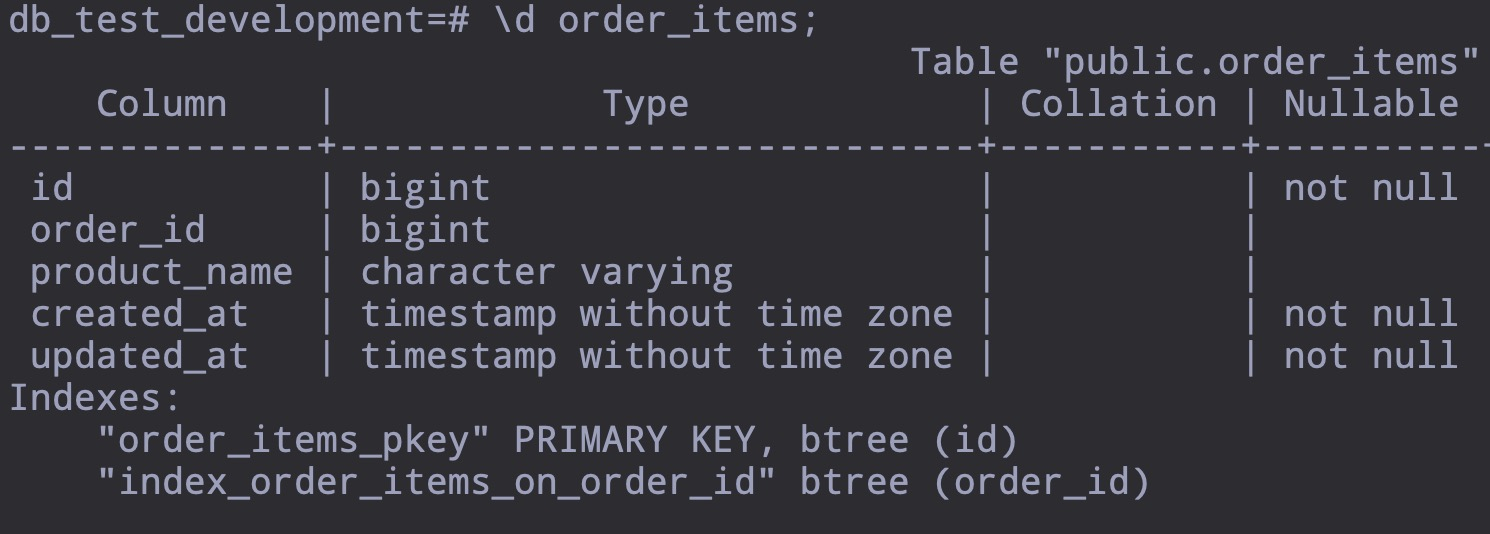• 一招秒杀 N+1 agg 问题 at 2018年11月11日

嗯 ，我忘了，我没有 deleted_at 字段，#21 的那里语句写的是按例子来的，实际的是下面这个

``````SELECT  orders.*, aggs.* FROM "orders" LEFT JOIN (SELECT order_id, COUNT(*) AS items_count FROM "order_items" GROUP BY "order_items"."order_id") AS aggs ON aggs.order_id = orders.id ORDER BY orders.id DESC LIMIT 10;
``````
• 一招秒杀 N+1 agg 问题 at 2018年11月11日

和索引无关，本地索引是对的order_items 的数据量为 20W+ orders 为 5W+

使用 LEFT JOIN LATERAL 在子查询里面多加 where 条件后好。感觉还是里面的查询不知道要使用 order_id 的索引来过滤数据，需要强制指定。如果没有这种用法，是不是就只能用子查询了？第一次见到这种写法又学了一招

• 一招秒杀 N+1 agg 问题 at 2018年11月10日
``````SELECT  orders.*, aggs.* FROM "orders" LEFT JOIN (SELECT order_id, COUNT(*) AS items_count FROM "order_items" WHERE "order_items"."deleted_at" IS NULL GROUP BY "order_items"."order_id") AS aggs ON aggs.order_id = orders.id LIMIT 10
``````

这个是正序的情况，是很快，但是 last 是带了 order by orders.id desc 的，好像速度不行~

``````SELECT  orders.*, aggs.* FROM "orders" LEFT JOIN (SELECT order_id, COUNT(*) AS items_count FROM "order_items" WHERE "order_items"."deleted_at" IS NULL GROUP BY "order_items"."order_id") AS aggs ON aggs.order_id = orders.id ORDER BY orders.id DESC LIMIT 10
``````

感觉在中间的子查询中还要再嵌套一层过滤 order_id 的，根据外层的条件来

不带 ORDER BY

``````Limit  (cost=0.71..6.73 rows=10 width=359) (actual time=0.072..0.127 rows=10 loops=1)
->  Merge Left Join  (cost=0.71..40027.18 rows=66537 width=359) (actual time=0.071..0.125 rows=10 loops=1)
Merge Cond: (orders.id = order_items.order_id)
->  Index Scan using orders_pkey on orders  (cost=0.29..9346.63 rows=66537 width=347) (actual time=0.028..0.054 rows=10 loops=1)
->  GroupAggregate  (cost=0.42..29731.93 rows=34768 width=12) (actual time=0.039..0.057 rows=5 loops=1)
Group Key: order_items.order_id
->  Index Only Scan using index_order_items_on_order_id on order_items  (cost=0.42..28311.68 rows=214514 width=4) (actual time=0.029..0.044 rows=21 loops=1)
Heap Fetches: 21
Planning time: 0.640 ms
Execution time: 0.244 ms
``````

带了 ORDER BY

``````Limit  (cost=12230.82..12232.33 rows=10 width=359) (actual time=254.210..254.258 rows=10 loops=1)
->  Merge Left Join  (cost=12230.82..22265.03 rows=66537 width=359) (actual time=254.208..254.253 rows=10 loops=1)
Merge Cond: (orders.id = order_items.order_id)
->  Index Scan Backward using orders_pkey on orders  (cost=0.29..9346.63 rows=66537 width=347) (actual time=0.025..0.059 rows=10 loops=1)
->  Sort  (cost=12230.53..12317.45 rows=34768 width=12) (actual time=254.177..254.179 rows=5 loops=1)
Sort Key: order_items.order_id DESC
Sort Method: quicksort  Memory: 3857kB
->  HashAggregate  (cost=8912.71..9260.39 rows=34768 width=12) (actual time=202.281..223.324 rows=53143 loops=1)
Group Key: order_items.order_id
->  Seq Scan on order_items  (cost=0.00..7840.14 rows=214514 width=4) (actual time=0.014..87.574 rows=214514 loops=1)
Planning time: 0.660 ms
Execution time: 255.422 ms
``````

如果在中间那个 group by 的子查询中再嵌套一个子查询带外层的条件，又感觉比较丑

•严重支持

• 看到下午茶进来的

• 网约车已经合法，OBD 还是挺热的，看好• 函数参数疑问 at 2016年08月20日

我的两个理解点 1，ruby 是浅拷备的 2，代码解释由右至左

a.push 的方法，基于浅拷备，不另辟内存空间，所以改变的是原值

add function 里面 a 是一个新变量会有一个新的 object_id，它只是指向 function 外面的 a 的地址，在 a = a 的过程只，只是声明了一个新的 object，并没有开辟内存空间去存值 a = a + b 先计算 a+b，然后指向新的内存地址

估计是为了省内存吧 乱猜的